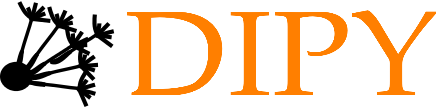## Statistical learning of human brain structure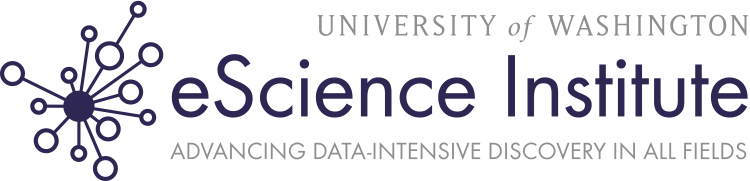### Normal behavior is supported by brain connectivity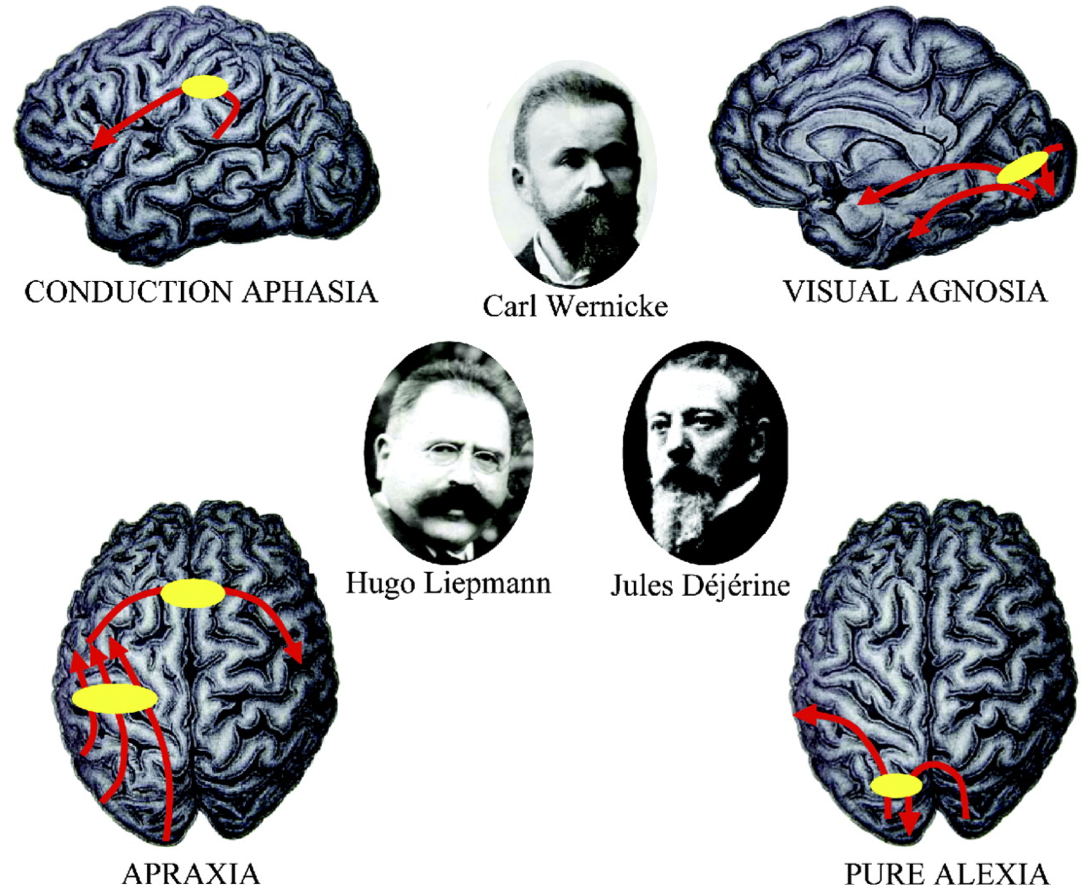Image from Catani and ffytche (2015)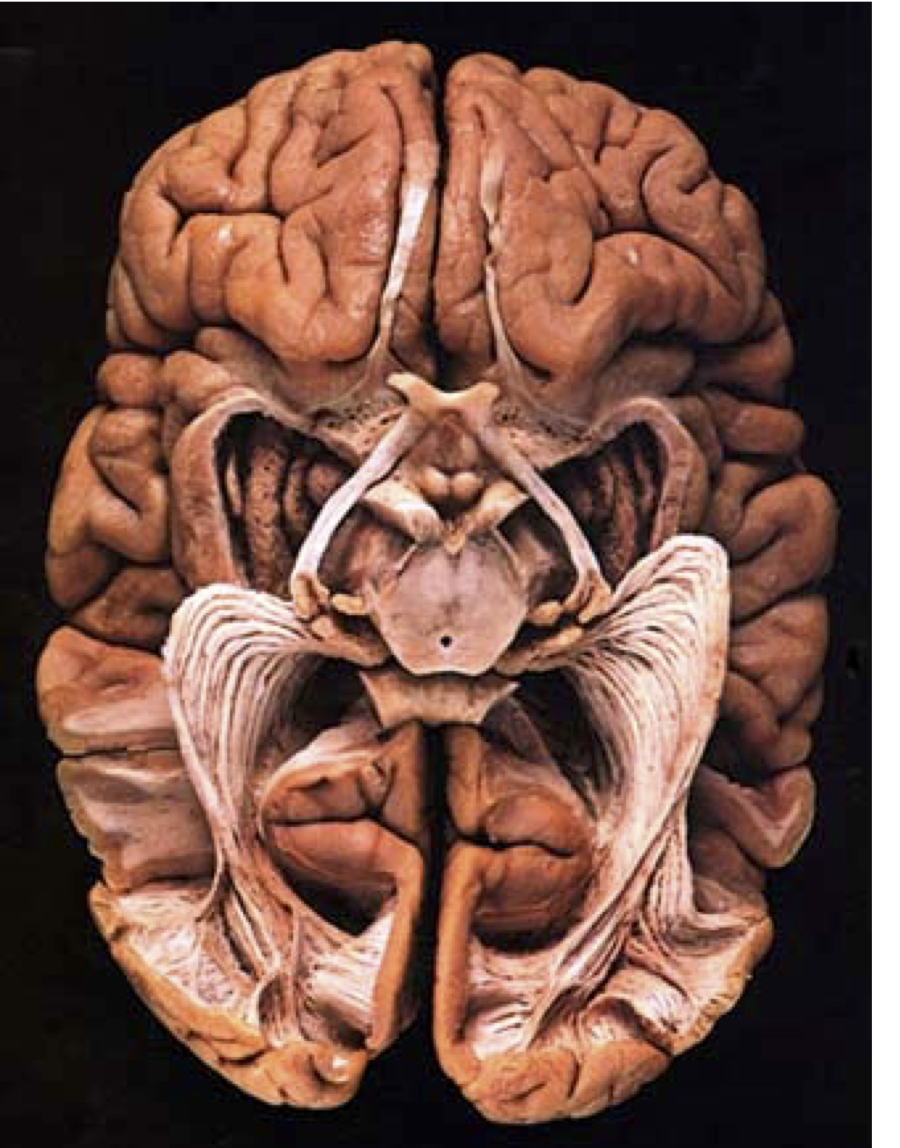## Not just passive cables

Brain connections change with development

Individual differences account for differences in behaviour

This has clinical significance

### Magnetic Resonance Imaging (MRI)

Neural activity: functional MRI

Anatomy: structural MRI

...

Brain connectivity: diffusion MRI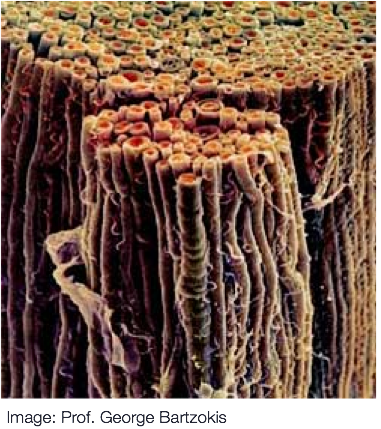## Diffusion MRI

Isotropic diffusion

## Diffusion MRI

Anisotropic diffusion## Diffusion MRI

### Modeling diffusionBasser, Mattielo and Le Bihan (1994)

### Diffusion statistics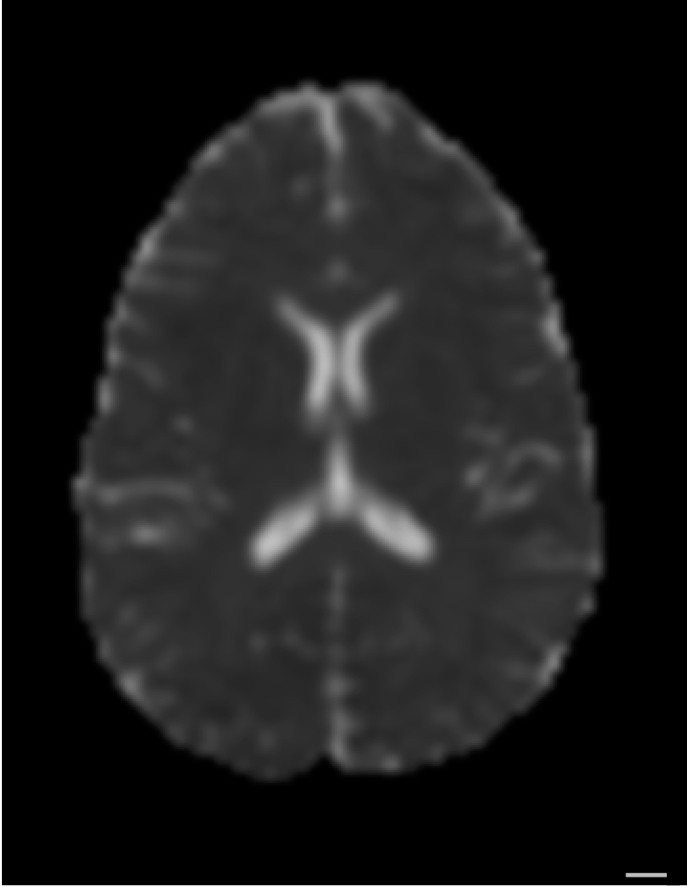Mean diffusivity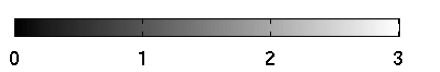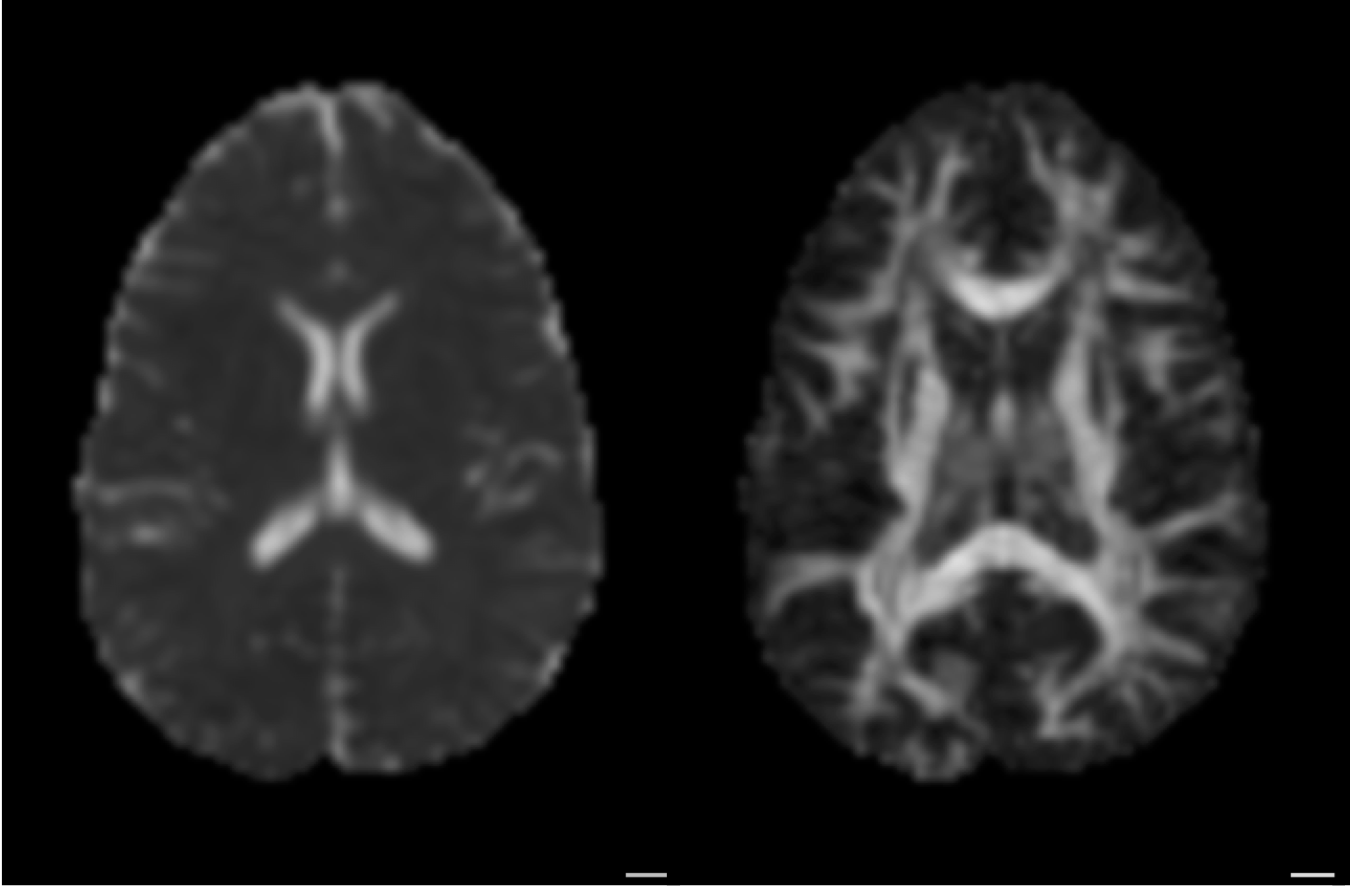Fractional anisotropy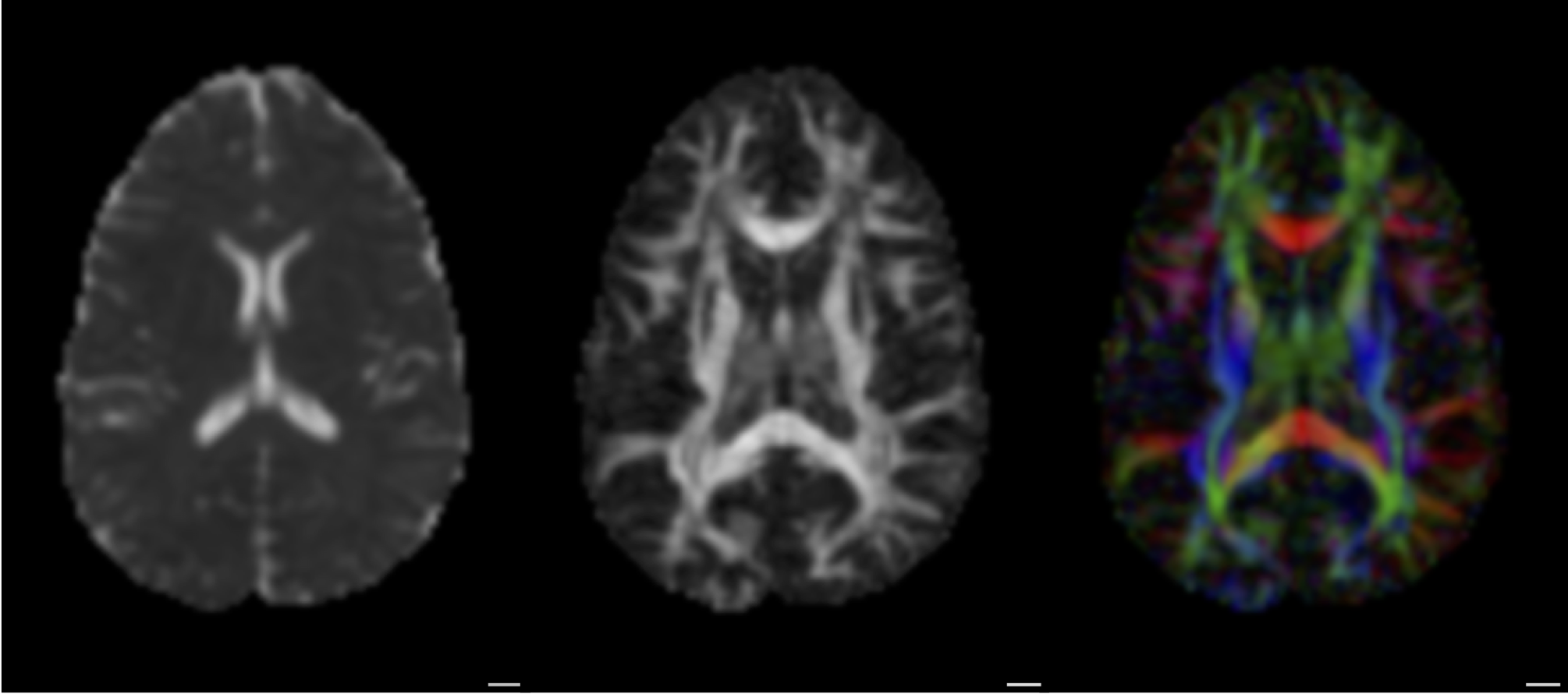Principal diffusion direction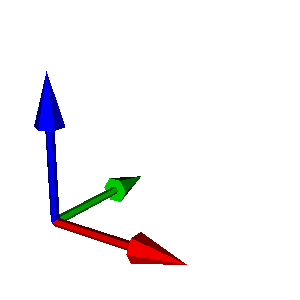### From diffusion to tracks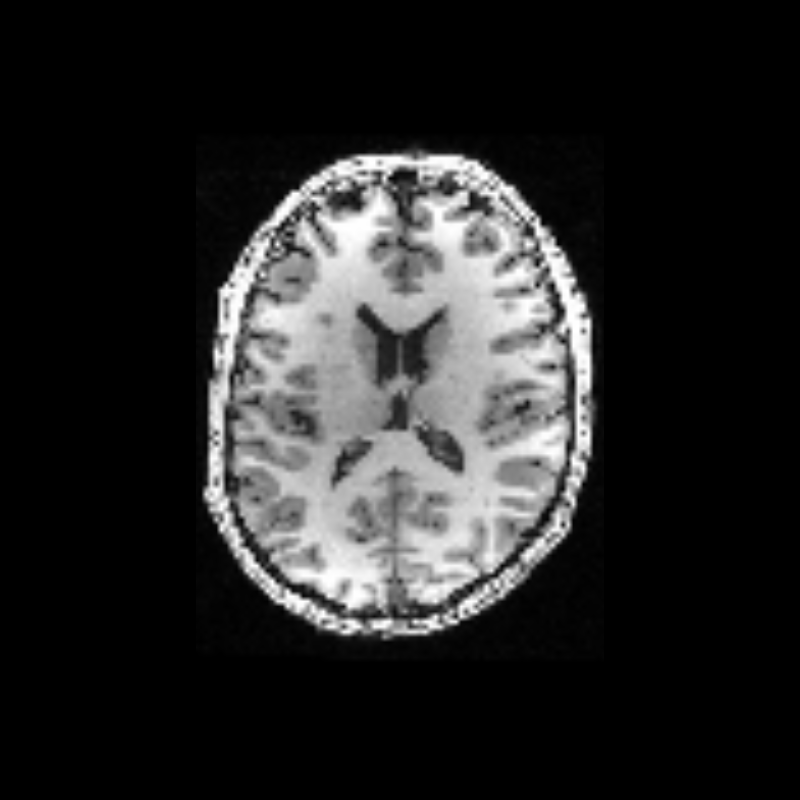### From diffusion to tracks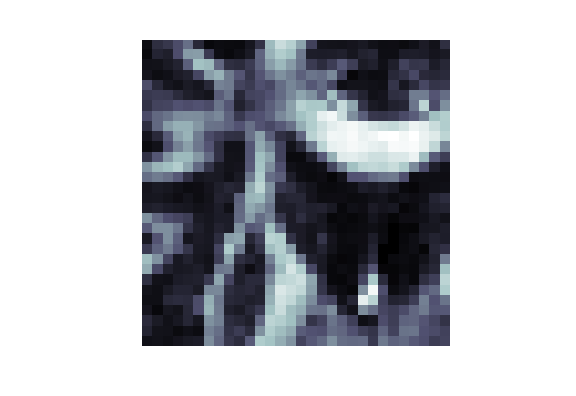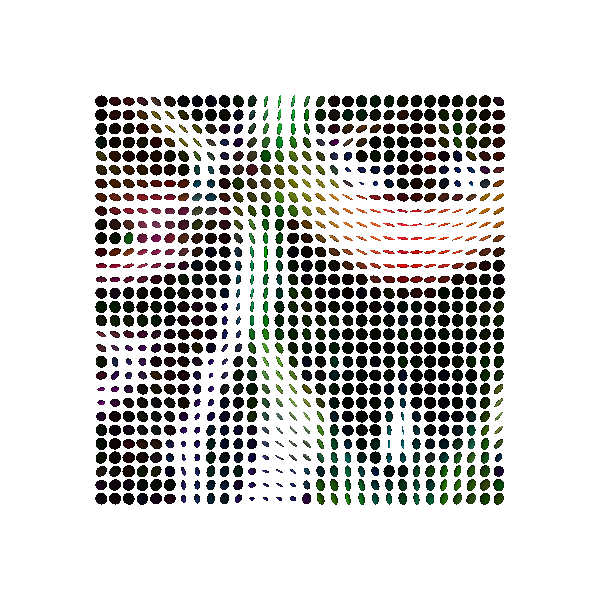## DIPY: Diffusion MRI in Python

Part of the NIPY community

Started in 2009 by Eleftherios Garyfallidis

Contributors from at least six different countries and many different labs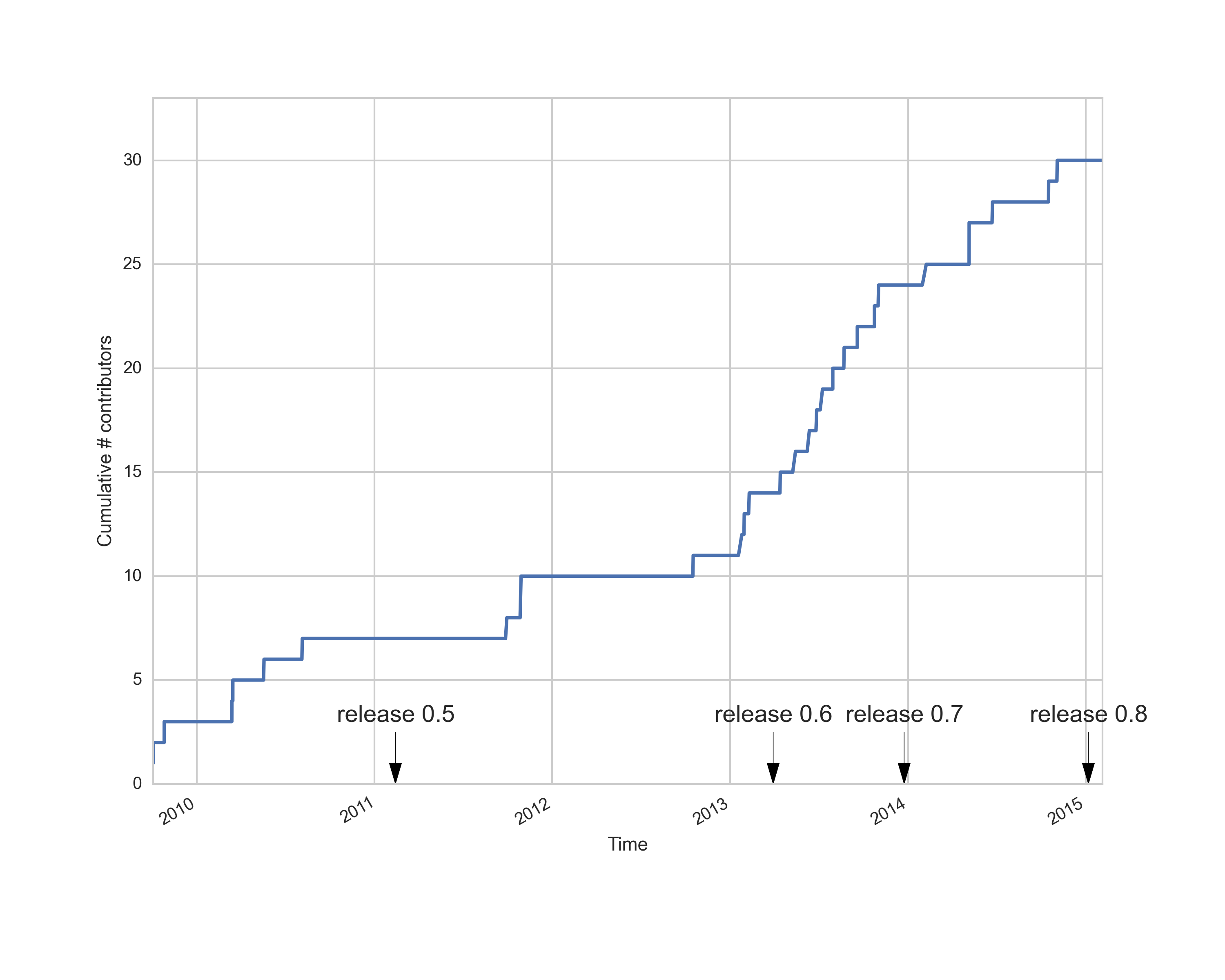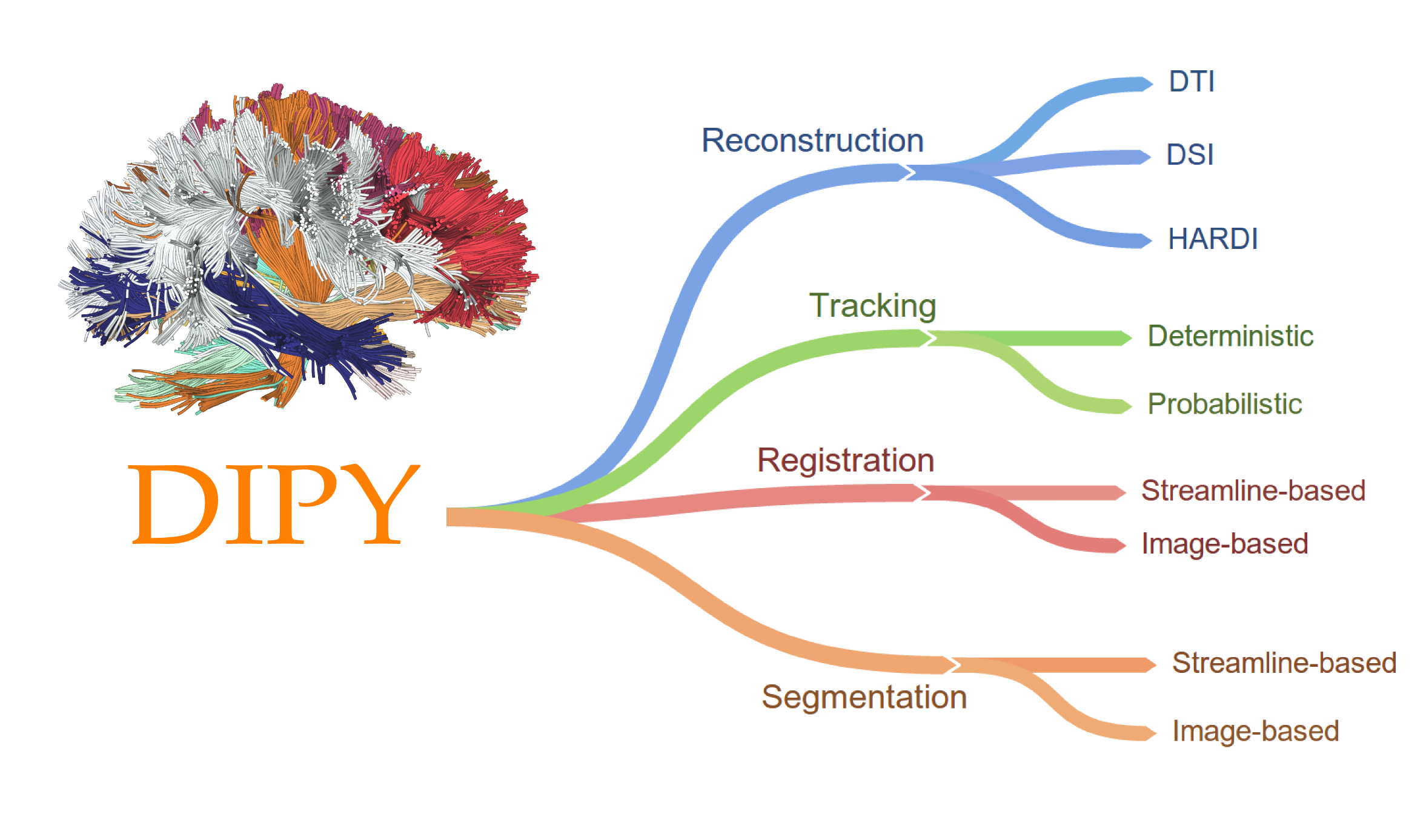### Diffusion MRI: the challenge of validation

Algorithm 1
Algorithm 2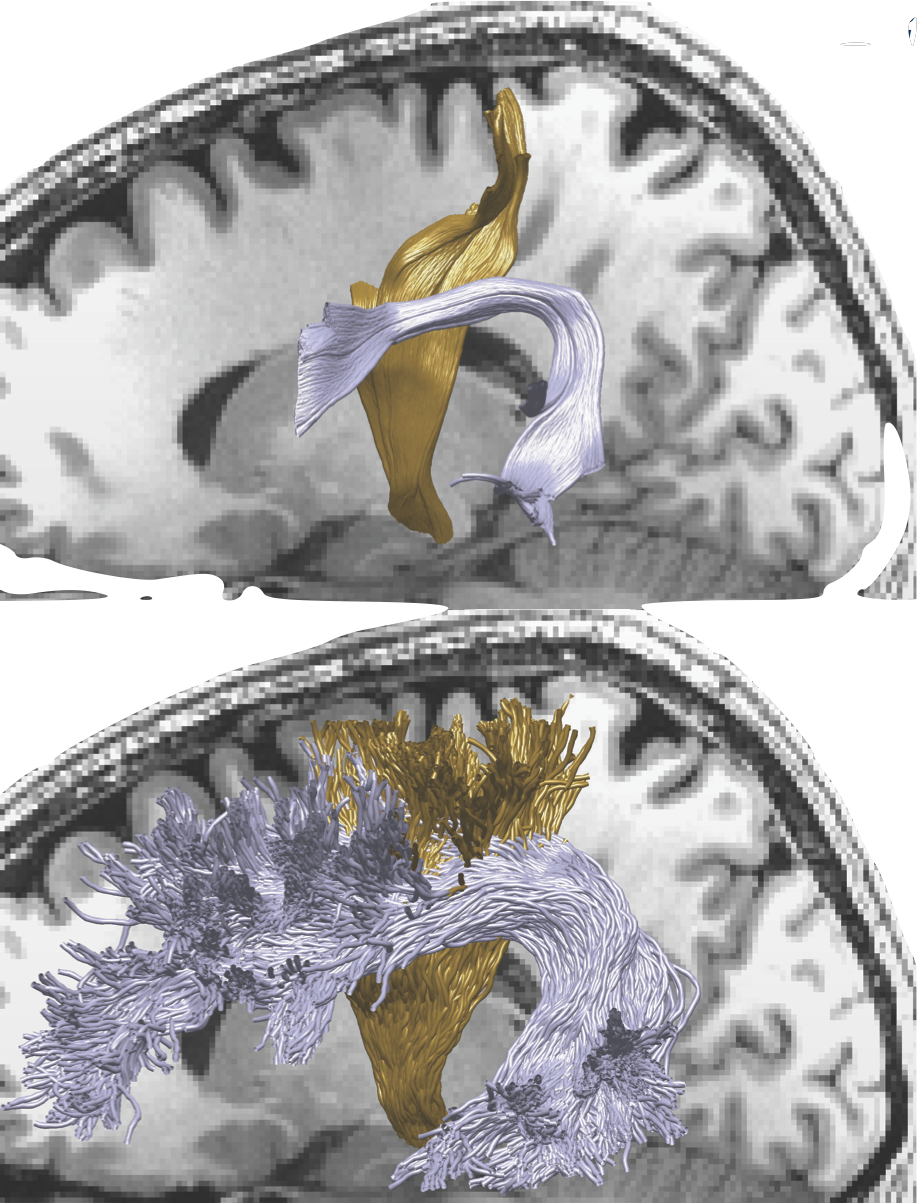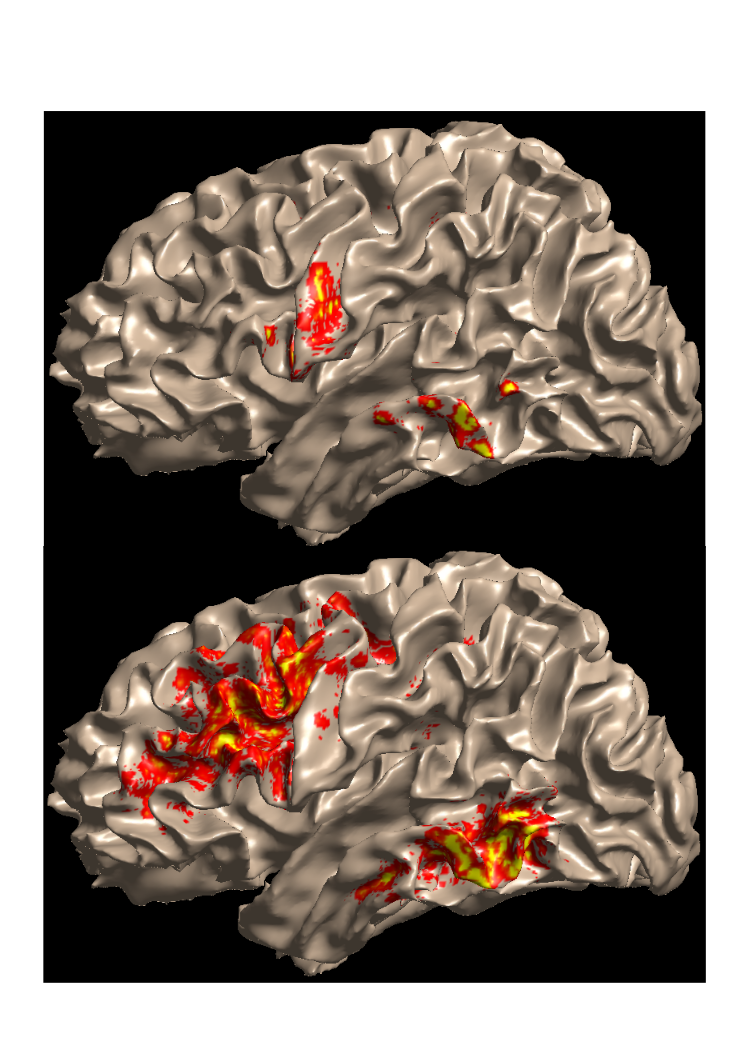## A statistical learning approach

In-vivo validation
Measurement #1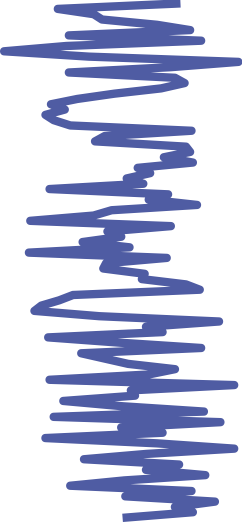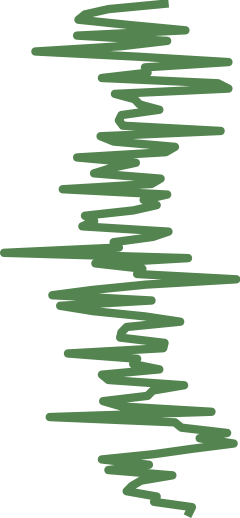Measurement #2
Test-retest reliability
Model
Cross-validation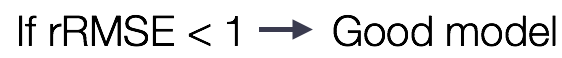Rokem et al. (2015)

## Dipy cross-validation API

``` ```
``` gtab = gradient_table(...) model = ReconstModel(gtab, ...) fit = model.fit(data, ...) # => ReconstFit prediction = fit.predict(gtab, ...) ```

## For example

``` ```
``` model = dti.TensorModel(gtab) fit = model.fit(data1) prediction = fit.predict(gtab) RMSE = np.sqrt(\ np.mean((prediction - data2) ** 2), -1)) ```

``` rRMSE = RMSE / np.sqrt(\ np.mean((data1 - data2) ** 2), -1)) ```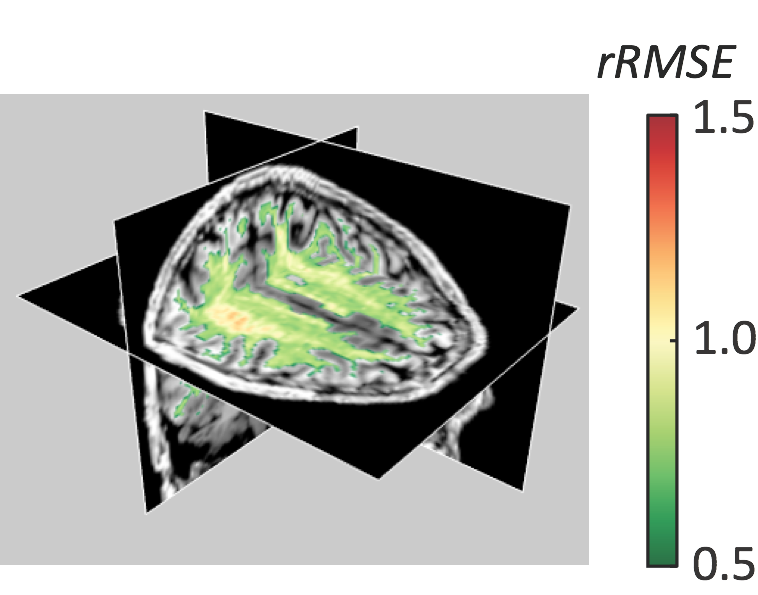Rokem et al. (2015)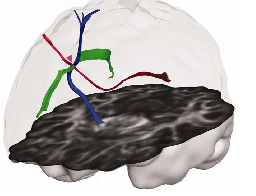Corpus callosum
Corticospinal tract
Superior
longitudinal fasciculus
DTICrossing fiber model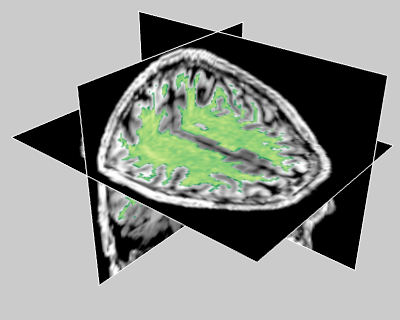Rokem et al. (2015)

### When you've only measured once

k-fold cross-validation ``` ```

``` # Use a k of 2 dti_pred = kfold_xval(dti_model, data, 2) ```

``` csd_pred = kfold_xval(csd_model, data, 2) ```Algorithm 1
Algorithm 2

## LiFE: Linear Fascicle Evaluation

Forward model from the tracks to the measured signal

Pestilli et al. (2014)

### From diffusion to tracks### From tracks to diffusion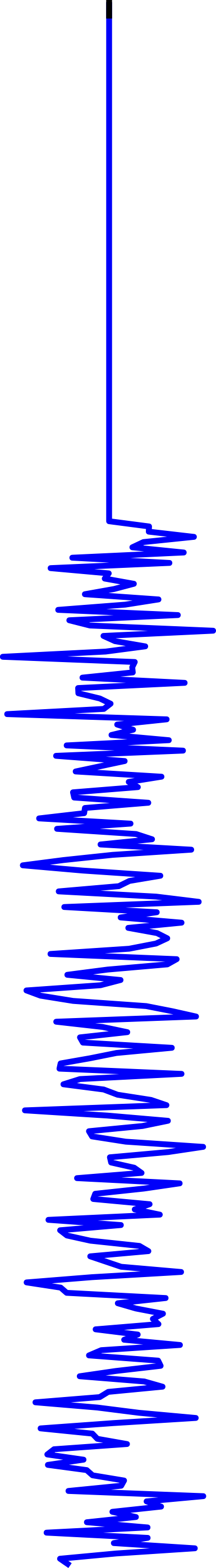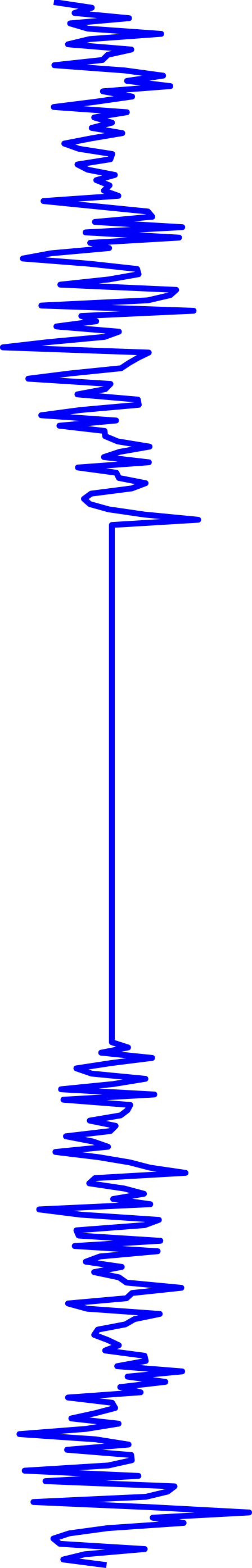...
=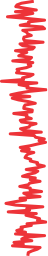Pestilli et al. (2014)Solve for``` >>> X.shape (10e8, 10e6) ```
Pestilli et al. (2014)
``` fiber_model = life.FiberModel(gtab) fit = fiber_model.fit(data, tracks) prediction = fit.predict(gtab) optimized_tracks = tracks[fit.beta>0] ```

## Summary

Measuring brain connectivity with diffusion MRI

The Dipy project

The validation problem

In vivo validation through statistical learning

## Collaborators

Dipy: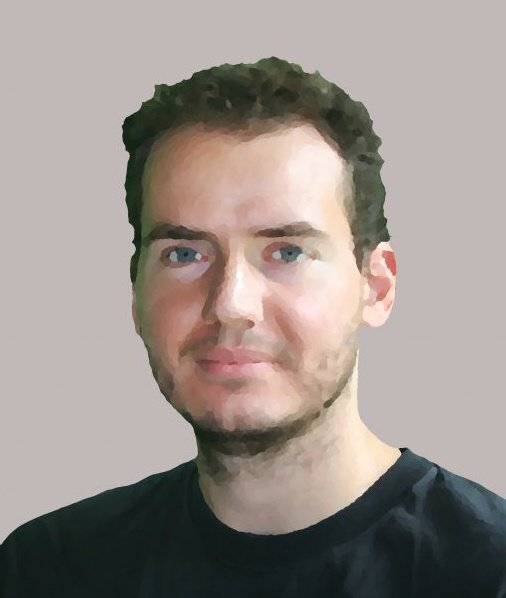Eleftherios
GaryfallidisStefan
Van der WaltBago
Amirbekian

## Collaborators

Stanford VISTA lab:Brian
Wandell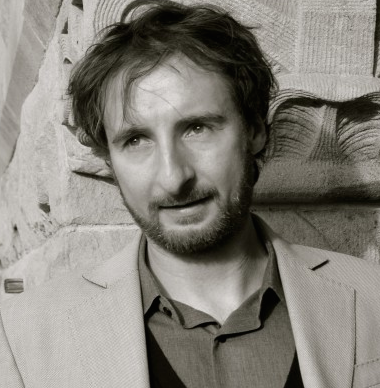Franco
Pestillihttp://arokem.orgarokem@gmail.com@arokemgithub.com/arokem